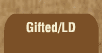Mathematics and Dyslexia

Tips for Learning the Times Tables

Tools for the Times Tables & Workbook

Home > Math > times tables practice >

## The Six Times Tables (all of them)

If you're getting tired of this... don't let up! There aren't that many more to go. Take the time to learn the tricky ones -- try all the tricks or make up your own.

1. 3 x 6=
2. 6 x 5=
3. 10 x 6=
4. 7 x 6=
5. 6 x 9=
6. 6 x 1=
7. 6 x 3=
8. 2 x 6=
9. 8 x 6=
10. 6 x 6=
11. 6 x 4=
12. 7 x 6=
13. 6 x 9=
14. 2 x 6=
15. 9 x 6=